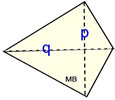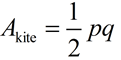Area & Perimeter of Familiar Polygons MathBitsNotebook.com Terms of Use   Contact Person: Donna Roberts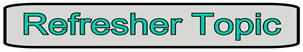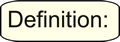Perimeter is the distance around the outside of a closed figure. It can be thought of as a "path" that surrounds a two-dimensional shape.

Shown below are some familiar perimeter formulas.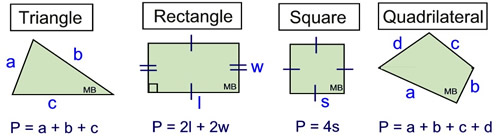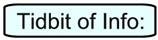When considering perimeter and area of a figure, is it true that as one gets larger, the other also gets larger?
 If you are dealing with an enlargement (a dilation) of a figure, increasing the area will also increase the perimeter. You cannot, however, generalize this result by stating that "increasing the area of a figure will always increase the perimeter of the figure". Consider the image at the right. The area of the pentagon is decreased by cutting chunks away, but the perimeter is increased.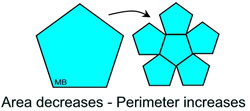Area is the quantity that expresses the amount of surface a two-dimensional shape covers in square units. The area of a shape can be measured by comparing the shape to squares of a fixed size.

Derivations of well known area formulas of 3 and 4-sided polygons:

Rectangle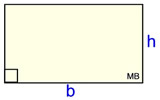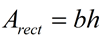Due to the perpendicular nature of rectangles, "unit squares" can be easily used to tile the rectangle's surface to represent the area. The unit squares can be subdivided to represent fractional lengths if needed. It can be observed that the area is found by multiplying the dimensions of the rectangle.

base b = a side of the rectangle
height h = a side that is perpendicular to the base
Parallelogram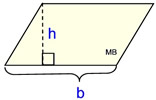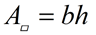A parallelogram can be thought of as a slanted rectangle. It can be dissected to create a triangle from the slanted portion which can be translated to the opposite side, creating a rectangle.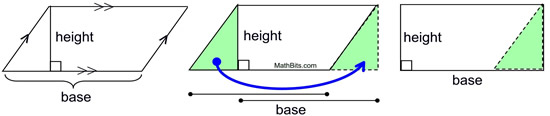Triangle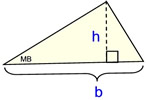The area of a triangle is related to the area of a rectangle. Consider 3 cases: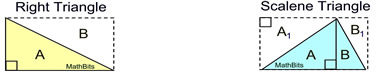ΔA congruent to ΔB area rectangle = areas ΔA + ΔB bh = area ΔA + area ΔA bh = 2(area ΔA) ½bh = area ΔA ΔA congruent to ΔA1; ΔB congruent to ΔB1 area rectangle = areas ΔA+ΔB+ΔA1+ΔB1 bh = areas ΔA+ΔB+ΔA+ΔB bh = 2(area ΔA+ΔB) ½bh = areas ΔA+ΔB = area given blue Δ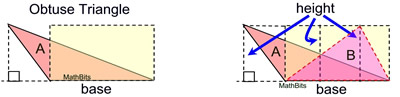Drawing a rectangle around triangle A will be counterproductive since the base of the triangle will no longer be the base of the rectangle. Instead, draw the rectangle to coincide the bases. The height of the triangle is the same as the height of the rectangle. Triangle B has the same area as triangle A (same base and height). We showed scalene triangles like B have area = ½bh.
Trapezoid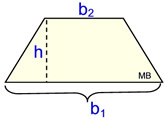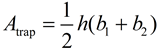A trapezoid is a composite figure made up of other geometric shapes. Partition the figure to form 2 triangles and one rectangle. area trap = area ΔA + area rect. C + area ΔB area trap = ½xh + b1h + ½yh = b1h + ½h(x + y) = b1h + ½h(b2 - b1) = b1h + ½b2h - ½ b1h = ½b1h + ½b2h = ½h( b1+ b2)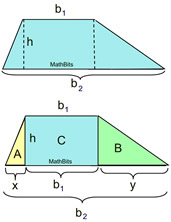Other formulas:
 Regular Triangle (Equilateral Δ )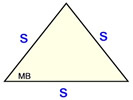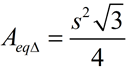Regular Quad (Square)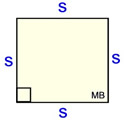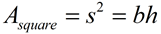Rhombus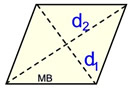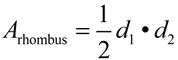Kite